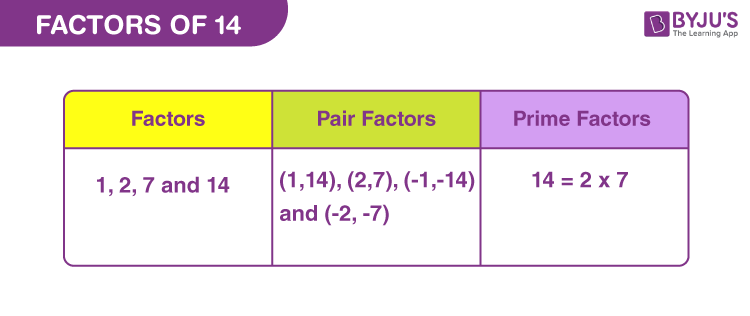# Factors of 14

Factors of 14 are those real numbers that can evenly divide the original number, without leaving any remainder. For example, 2 is the factor of 14 because when we divide 14 by 2, we get a whole number, such as;

14 divided by 2 ⇒ 14 ÷ 2 = 7

In the same way, we can find the other factors of 14 here in this article. Also, we will come across the pair factors of 14, that produces the original number upon multiplication with each other. Prime factors of a number are the prime numbers that can evenly divide the original number.## How to Find Factors of 14?

Factors of 14 are those natural numbers that can completely divide the original number, without leaving any remainder. We will start dividing 14 by the smallest natural number and continue dividing it by 14.

14 ÷ 1 = 14 [Whole number]

14 ÷ 2 = 7 [Whole number]

14 ÷ 3 = 4.67 [Fraction]

14 ÷ 4 = 3.5 [Fraction]

14 ÷ 5 = 2.8 [Fraction]

14 ÷ 6 = 2.33.. [Fraction]

14 ÷ 7 = 2 [Whole number]

Now if we further divide 14 by 8, 9, 10, 11, 12 and 13, the quotient will be a fraction only.

At last divide 14 by itself, to get a quotient equal to 1.

14 ÷ 14 = 1 [Whole number]

Thus, we can conclude that only 1, 2, 7 and 14 are the factors of 14.

## Pair Factors of 14

To find the pair factors of 14, multiply the two numbers, to get the original number as 14.

1 × 14 = 14

2 × 7 = 14

Therefore, the pair factors are (1, 14) and (2, 7). These are the positive pair factors.

Similarly, we can also consider negative pair factors. When we multiply two negative factors, it will result in a positive value.

-1 × -14 = 14

-2 × -7 = 14

Therefore, the negative pair factors are (-1, -14) and (-2, -7).

## Prime Factorisation of 14

To find the prime factors of 14 we need to divide it by each of the prime numbers till the remainder is 1.

Step 1: Divide 14 by the smallest prime factor, which is 2.

14/2 = 7

Step 2: Again divide 7 by the next prime factor that is 7 itself. (Since 2 and 3 are smaller than 7).

7/7 = 1

Step 3: We cannot divide 1 by any prime factor, further. Therefore,

 Prime factorisation of 14 = 2 x 7

## Solved Examples

Q.1: If there are 14 apples to be distributed among 7 members in a room. How many apples does each member get?

Solution: Given,

Number of apples = 14

Number of members in room = 7

Each member will get = 14/7 = 2 apples

Q.2: What is the sum of all the factors of 14?

Solution: The factors of fourteen are 1, 2,7 and 14.

Sum = 1 + 2 + 7 + 14 = 24

Therefore, 24 is the required sum.

Q.3: What are the common factors of 7 and 14?

Answer: To find the common factors, we need to write down the factors of each of the given numbers.

7 is a prime number, has only two factors.

7 → 1 and 7

14 is a composite number, hence has more than two factors.

14 → 1, 2, 7 and 14

Hence, the common factors among 7 and 14 are 1 and 7.

## Frequently Asked Questions on Factors of 14

### What are the total factors of 14?

The factors of 14 are 1, 2, 7 and 14. Hence, in total there are four factors.

### 14 is a multiple as well as factor of 14. True or false?

True. 14 is both factor and multiple of natural number 14, because 14 divided by 14 is equal to 1 and inversely, 14 multiplied by 1 is 14.

### What are the multiples of 14?

The first 10 multiples of 14 are 14, 28, 42, 56, 70, 84, 98, 112, 126 and 140.

### What are the prime factors of 14?

The prime factorisation of 14 is equal 2 x 7. Therefore, 2 and 7 are the prime factors of 14.

### What is the average of factors of 14?

Average is the sum of all the factors divided by a number of factors.
Average (factors of 14) = (1+2+7+14)/4 = 24/4 = 6
Therefore, the required average is 6.